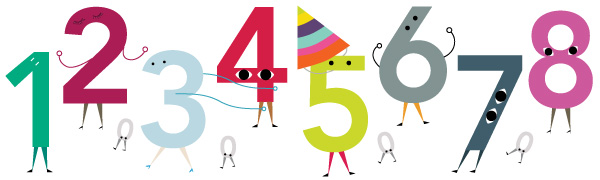Do They Satisfy? (Part 1)For the next few problems of this series, you will be given a property $P$ and a bunch of (usually $8$) numbers or expressions. Your answer will be an $n$-digit number, where $n < 10$ is the number of numbers or expressions given. For the $k$-th number or expression, if it satisfies property $P$, then on the $k$-th digit of your answer, enter $k$. If not, enter $0$ as the $k$-th digit. For example, if $P$ : even, and there are $3$ numbers, $4, 7, 3182$. Then, enter your answer as $103$.

$P$ : primes

1. $2$

2. $65537$

3. $2999999$

4. $4999999$

5. $98320414332827$

6. $2^{35} - 1$

7. $34567875111$

8. $12345678987654321$

You can try Part 2 and Part 3.

×